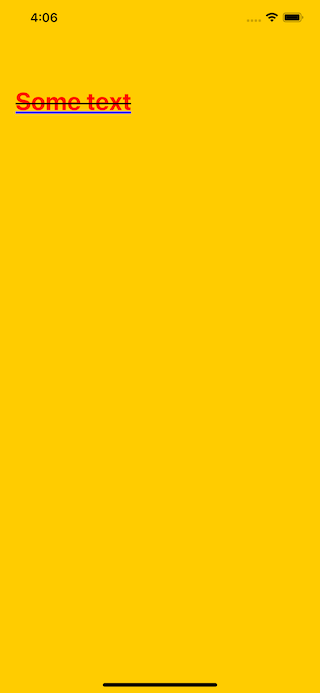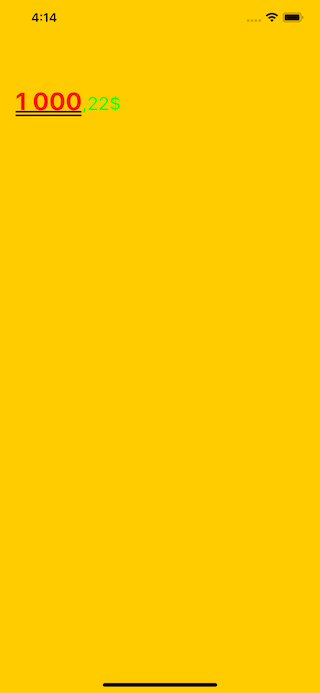# Extendy

A set of useful string extensions.

• iOS 11.0+
• Swift 5+

## Installation

### CocoaPods

Extendy is available through CocoaPods. To install it, simply add the following line to your Podfile:

``````pod 'Extendy', '~> 1.1'
``````

### Swift Package Manager

The Swift Package Manager is a tool for automating the distribution of Swift code and is integrated into the `swift` compiler.

Once you have your Swift package set up, adding Extendy as a dependency is as easy as adding it to the `dependencies` value of your `Package.swift`.

``````dependencies: [
.package(url: "https://github.com/NovichenkoAnton/Extendy.git", .upToNextMajor(from: "1.1"))
]
``````

## Usage

### String

1. Apply masks for the string in specific range. The range compatible with `CountableRange`, `ClosedRange`, `PartialRangeFrom`, `PartialRangeThrough`, `PartialRangeUpTo`.
``````let cardNumber = "1234567890123456"
``````
1. Convert `String` to `Double`. If `String` is not compatible with `Double` the function will return 0.00.
``````let amount = "100,12"
print(amount.toDouble()) //100.12

let anotherAmount = "1 200,10"
print(anotherAmount.toDouble()) //1200.1
``````
1. You can apply specific format for strings
``````let sum = "1234"
let formattedSum = sum.ext.applyFormat(.sum)
print(formattedSum) //"1 234,00"
``````

Supported formats

``````enum Format {
case sum(minFractionDigits: Int = 2, maxFractionDigits: Int = 2)
case creditCard
case iban
case custom(formatter: NumberFormatter)
}
``````
1. Validate a number of credit card by Luhn algorithm.

2. Validate the string with specific pattern

``````"https://www.google.com".validate(with: .website) //true
``````
1. Simple date formatter (from one format to another)
``````let dateTime = "2019-11-22 13:33"

let resultTime = dateTime.ext.convertDate(from: "yyyy-MM-dd HH:mm", to: "h:mm") //"1:33"
``````
1. Get query items from `String` that corresponds to `URL` type. Works for URLs with cyrillic domain names.
``````let stringURL = "https://test.com?foo=1&bar=abc"

let queryItems = stringURL.queryItems()! //["foo": "1", "bar": "abc"]
``````

### NSMutableAttributedString

1. You can append two attributed strings with `+`
``````let part1 = "123"
let part2 = "456"

myLabel.attributedText = part1.attributed + part2.attributed
``````
1. Apply attributes for mutable string
``````let string = "Some text"

label.attributedText = string.attributed.applyAttributes([
.color(color: .red),
.font(font: .systemFont(ofSize: 32, weight: .bold)),
.crossed(width: 1, color: .black),
.underline(style: .single, color: .blue)
])
``````1. Apply styles for string
``````let sum = "1000,22"

label.attributedText = sum.attributed.applyStyle(.sum(integerAttrs: [
.color(color: UIColor.red),
.font(font: UIFont.systemFont(ofSize: 32, weight: .bold)),
.underline(style: .double, color: .black)
], fractionAttrs: [
.color(color: UIColor.green),
.font(font: UIFont.systemFont(ofSize: 24, weight: .medium)),
], currencyMark: "\$"))
``````## GitHub

https://github.com/NovichenkoAnton/Extendy